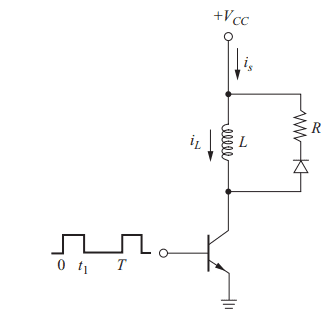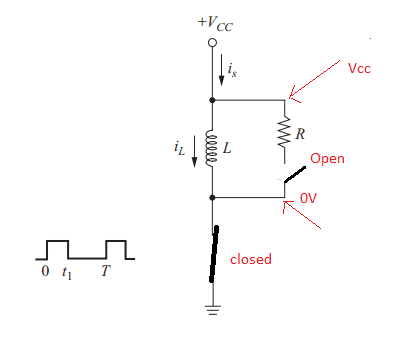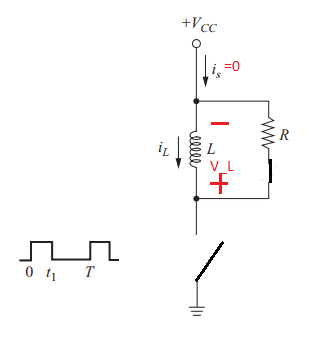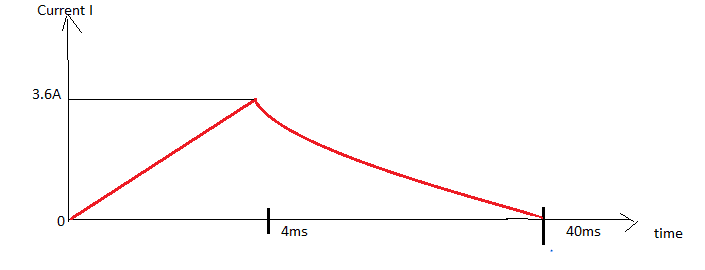# Peak energy stored in inductor

• PhysicsTest
I think the resistor is just keeping the voltage across the inductor at a constant level. I don't think the resistor is just keeping the voltage across the inductor at a constant level.

#### PhysicsTest

Homework Statement
An inductor is energized as in the circuit shown in fig. The circuit has L =100 mH, R= 20 ohm, VCC= 90 V, t1= 4 ms, and T= 40 ms. Assuming the transistor and diode are ideal, determine (a) the peak energy stored in the inductor, (b) the energy absorbed by the resistor in each switching period, and (c) the average power supplied by the source. (d) If the resistor is changed to 40 ohm, what is the average power supplied by the source?
Relevant Equations
P = V*I Watts; V_L = Ldi/dt
The figure is as shown belowa. From 0< t < t1 => 0 <t<4ms, the transistor is "ON"

The circuit will become like thisThe current ##i_s = i_L##. To know the magnitude of the current ##i_L## do i need to know the impedance of the inductance or since it is DC voltage the impedance is not applicable? The energy stored will then be ##E=\frac{LI^2} 2##.
The circuit during t1<t < T => 4<t<40ms will beThere is a voltage ##V_L## developed across the inductor because of the previous cycle and hence it forward biases the diode. There is no current flow through the source as the transistor is off. The inductor discharges through the resistor. My problem is how to use the equations and find the voltages and currents. Please help.

•Delta2
I think you have analyzed it correctly. For your first question when the switch is closed, you absolutely need to take into account the inductive reactance of the inductor, otherwise the current would be infinite since an ideal inductor has no resistance. Can you write a differential equation for the current I in this case? You should know the equation for the current through an inductor. Similarly, you need to write a differential equation for the current I in the second case when the switch is open.

•PhysicsTest
Ok, i got the idea i need to apply the KCL and KVL equations. For the circuit of fig1 when the transistor is closed
##V_{CC} = V_L##
##V_{CC} = L\frac{di} {dt}##
##di = \frac{V_{CC}} {L} dt##
##i =\frac{V_{CC}} L t## ->eq1
The energy stored in the inductor is
##E = \frac 1 2 Li^2## ->eq2
From eq1 and substituting the values the max current "i" is
##i = \frac{90*4*10^{-3}} {100*10^{-3}} A##
##i =3.6A##
Using eq2 to find the energy is
##E = \frac{100*10^{-3} * 3.6^2} {2} = 0.648 J##
Am I going in correct direction?

Yes, I think so. Keep going. What happens when the switch is opened?

When the switch is opened
##V_L = -V_R##
##L\frac{di_L} {dt} = -i_L R##
##\frac{di_L} {i_L} = \frac{-R} {L} dt## Integrating on both sides
##\int{\frac{di_L} {i_L}} = \int{\frac{-R} {L} dt}##
##\ln(i_L) = \frac{-R} {L} t + C## -> eq21
At t=t1=4ms from the previous calculation ##i_L = 3.6A ## Hence eq21 becomes
##\ln(i_L) = \frac{-R} {L} t + 3.6##
##i_L = e^{ \frac{-R} {L} t + 3.6}## ->eq22
substituting the values of R and L the eq22 becomes
##i_L = e^{-200t + 3.6} A ##
The energy observed by resistor is ## dE = i^2Rdt##
##E = \int_{4*10^{-3}}^{40*10^{-3}} e^{-400t+7.2} dt## ->eq23
##E = [e^{400t-7.2}]_{4*10^{-3}}^{40*10^{-3}} J##
##E = 6.6*10^3 J## ->eq24
I am not sure some of the equations are correct or not?
c. The average power supplied by source is
##P_{Avg} = \int_0^{40*10^{-3}}{V_{CC} * i(t)dt}##
##P_{Avg} = \int_0^{4*10^{-3}} V_{CC} * \frac{V_{CC}} Lt dt##
##P_{Avg} = [\frac{{V_{CC}}^2 Lt^2} 2]_0^ {4*10^{-3}} W##
##P_{Avg} = 6mW ##
d. The average power remains unchanged if the resistor changed to 40 Ohm

I think you have done the integration correctly, but I don't think you have calculated the constant of integration correctly. Try sketching out the current through the inductor as a function of time. As far as the energy, where does the energy dissipated in the resistor come from? This will give you a good cross-check. Your energy and power numbers cannot be correct. If ~6000J gets dissipated in the resistor each 400mS, how can the total power only be 6mW?

PhysicsTest said:
##\ln(i_L) = \frac{-R} {L} t + C## -> eq21
.
##\ln(i_L) = \frac{-R} {L} t + 3.6##
Logarithms are dimensionless (no units). So to have an equation with a term such as ‘##ln(i_L)##’ and another term ‘3.6’(amps) can’t be dimensionally correct. The equation would be inhomogeneous. So your constant, ‘C’, is not a current (not 3.6).

Here are a few other thoughts…

I don't understand why 3.6A (the current through L at the end of the ‘charging’ phase) should be the same as the current through R at the start of the ‘discharge’ phase. But I'd need to think about it.

There’s another (simpler) approach to part b):
1) Work out the time constant (L/R) for the ‘discharge’ phase.
2) Compare this to the avaulable ‘discharge’ time till the start of the next cycle.
3) What can you deduce?

I will also note this is a poor question because (as far as I can see) L doesn't fully ‘discharge’ through R each cycle. Over many cycles this would become significant. This make a rigorous analysis harder. But I think the person who set the question intended you to ignore this (or didn't consider it).

Steve4Physics said:
I will also note this is a poor question because (as far as I can see) L doesn't fully ‘discharge’ through R each cycle. Over many cycles this would become significant. This make a rigorous analysis harder. But I think the person who set the question intended you to ignore this (or didn't consider it).
I don't think it is that bad of a question. The discharge time is 7 time constants. exp(-7) = 0.0009, so for all practical purposes the inductor current has discharged, and I suspect this is what the person who wrote the question wants you to assume. Making reasonable approximations is part of the learning process.

phyzguy said:
I don't think it is that bad of a question. The discharge time is 7 time constants. exp(-7) = 0.0009, so for all practical purposes the inductor current has discharged, and I suspect this is what the person who wrote the question wants you to assume. Making reasonable approximations is part of the learning process.
Yes, I was being a bit harsh. And I definitely agree that learning to make reasonable approximations is part of the learning process (which is why I suggested considering the time constant!).

It’s also worth being aware of the limitations of an approximation. Over a large number of cycles, there will be a cumulative error introduced by using the ‘full discharge’ approximation. T = 40ms gives 90,000 cycles per hour. Parts c) and d) are about ‘average’ power - the average power in the early part of a 1 hour period would (I suspect) be significantly different to the average power near the end. Hence my criticism.

This question is a steady state problem. Yes you can, and perhaps should solve the equations in the two states. But then if you go that route you have to match up the initial conditions in each period to insure that the inductor current is continuous and isn't changing, on average, from cycle to cycle.

The change of the inductor current is given by (1/L)⋅∫v⋅dt over the whole cycle. In steady state the integral equals zero when integrated over exactly one cycle, because the net current change should be zero. This allows you to derive the "volt-second" balance criteria for steady state switched inductor circuits. This isn't as hard as it may sound. A common and important assumption is that the L/R time constant is large compared to the switching time, which allows you to make a linear approximation for the shape of the inductor current.

So, you want to consider a two step process for this solution. First what is the average inductor current (the steady state solution). Then what is the peak deviation during a single switching cycle.

If you want to look into this on the web search for "buck power supply analysis". That should give you some good links.

edit: Oops! I just noticed that the "fast switching" assumption doesn't apply. The relaxation time of 36msec is 7.2*L/R. So the one cycle analysis is quite close to the steady state solution (99.93% discharged). I'll leave my post, but it is only applicable to this problem in a pedantic sense.

Last edited:
Yes i understood the mistake with the constant of integration, the current will look something like this.The constant of integration is

##\ln(i_L) = \frac{-Rt} {L} + C## ->eq31
if t = 4ms ##i_L=3.6A## substitute in eq31
##\ln(3.6) = \frac{-20*4*10^{-3}} {0.1} + C##
##1.28 = -0.8 + C => C = 2.08##
Hence ##i_L = e^{-200t + 2}##
The energy
##E = \int_{4*10^{-3}}^{40*10^{-3}} e^{-400t+4} * 20 dt ##
##E = \frac{20} {-400}[e^{-400t + 4}]_{4*10^{-3}}^{40*10^{-3}} ##
##E = 0.551 J ##. I don't know how to cross check.

That is correct except that as @DaveE points out in steady state the graph is shifted slightly up and the current never quite returns to zero. This offset is small and stable (not increasing as @Steve4Physics contends).

•Steve4Physics
hutchphd said:
That is correct except that as @DaveE points out in steady state the graph is shifted slightly up and the current never quite returns to zero. This offset is small and stable (not increasing as @Steve4Physics contends).
I stand corrected. I’m probably mis-thinking because of the unusual (to me) ‘charging’ process with constant voltage and zero resistance. ` I’ll give it some more thought.

•hutchphd
PhysicsTest said:
Yes i understood the mistake with the constant of integration, the current will look something like this.
View attachment 278476
The constant of integration is

##\ln(i_L) = \frac{-Rt} {L} + C## ->eq31
if t = 4ms ##i_L=3.6A## substitute in eq31
##\ln(3.6) = \frac{-20*4*10^{-3}} {0.1} + C##
##1.28 = -0.8 + C => C = 2.08##
Hence ##i_L = e^{-200t + 2}##
The energy
##E = \int_{4*10^{-3}}^{40*10^{-3}} e^{-400t+4} * 20 dt ##
##E = \frac{20} {-400}[e^{-400t + 4}]_{4*10^{-3}}^{40*10^{-3}} ##
##E = 0.551 J ##. I don't know how to cross check.
In post #3 you (correctly I think) calculated the energy in the 'charged' inductor to be 0.684J.

Have you worked out the discharge time-constant (##\tau = L/R##) yet? Worth doing.

From previous posts mentioning the time-constant during 'discharge', I hope you have realized that virtually all of the inductor’s energy is dissipated in the resistor, each cycle. So to 'cross-check', you can compare your value of 0.551J to 0.684J. They don’t match – so something’s wrong.

I can’t follow your working easily. I’d treat the ‘discharge’ as a totally separate process. So during discharge, t runs from t=0 to t=36ms, rather than t=4ms to t=40ms. And you know I=3.6A at t=0. That keeps the working simpler.

The energy stored in the inductor
##E = \int_0^{4*10^{-3}} v(t)i(t) dt##. Using eq1 ##i(t) = \frac{V_{CC}t} {L}##
##E = \frac 1 L \int_0^{4*10^{-3}}V_{CC}^2t dt ##
##E = \frac {V_{CC}^2} {2L} [t^2]_0^{4*10^{-3}} ##
substituting ##V_{CC} = 90V, L = 0.1 H ##
##E = \frac{(90)^2*16*10^{-6}} {0.2} J = 0.648J##. I tried to calculating the average energy, but i am surprised that it matches with the energy calculated with peak current? Is it always the case? The current is varying hence i cannot take a constant (peak) value to calculate energy? Does it mean the average energy and the peak energy in the inductor are always same?
I will start working on the discharge time constant.

@PhysicsTest I think your graph is qualitatively correct, except it discharges much faster than what you have shown. As @Steve4Physics has said, basically all of the energy stored in the inductor gets dissipated in the resistor each cycle. In terms of the average, remember that you were asked for the average power, not the average energy.

PhysicsTest said:
The energy stored in the inductor
##E = \frac{(90)^2*16*10^{-6}} {0.2} J = 0.648J##.
OK. That's the total energy stored after 4ms.
PhysicsTest said:
I tried to calculating the average energy,
Don’t understand. Which type energy (magnetic or heat) do you mean? And over what time-period is the average taken?
Anyway, part b) is to calculate how much energy is turned to heat during 'discharge' through the resistor. 'Average energy' is not useful/relevant, And as already pointed out by @phyzguy, part c) and d) require average power.
PhysicsTest said:
I will start working on the discharge time constant.
Shouldn’t take too long to divide one number by another! Then you can answer part b) immediately.